# i want to verify my answer because i am not sure. i got 13.70932267 2 H2...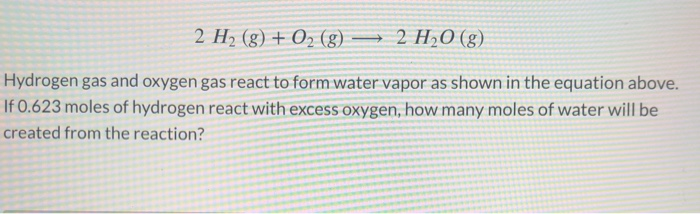i want to verify my answer because i am not sure. i got 13.70932267
2 H2 (g) + O2 (g) — 2 H2O(g) Hydrogen gas and oxygen gas react to form water vapor as shown in the equation above. If 0.623 moles of hydrogen react with excess oxygen, how many moles of water will be created from the reaction?

Given

2H2(g) + O2 (g)= 2 H2O(g)

According to the above balanced equation

2 moles of H2 reacts with excess of O2 to form 2 moles of H2O

Therefore 0.623 moles of H2 with excess of O2 will form = 2/2 X 0.623 moles of H2O

= 0.623 moles of H2O

#### Earn Coin

Coins can be redeemed for fabulous gifts.

Similar Homework Help Questions
• ### I am a student in the ninth grade and I need help with the following problems because I do not understand how to do them

I am a student in the ninth grade and I need help with the following problems because I do not understand how to do them. I appreciate your help and thanks once again. 7. in 2CO(grams) + O2(grams) => 2CO2(grams), what is the ratio of moles of oxygen used to moles of CO2? 9. when iron rusts in air, iron(III) oxide is produced. how many moles react with 2.4 iron in the rusting reaction? 4Fe(s) + 3O2(g) => 2Fe2O3(s) 22....

• ### How many moles of methane are produced when 25.1 moles of carbon dioxide gas react with excess hydrogen gas?

Hi! So my homework gives this information, "Under certain circumstances, carbon dioxide, CO2(g), can be made to react with hydrogen gas, H2(g), to produce methane, CH4(g), and water vapor, H2O(g): CO2(g)+4H2(g)→CH4(g)+2H2O(g)" I need explanations on how to do these two problems: 1.) How many moles of methane are produced when 25.1 moles of carbon dioxide gas react with excess hydrogen gas? 2.) How many moles of hydrogen gas would be needed to react with excess carbon dioxide to produce 19.1...

• ### Under certain circumstances, carbon dioxide, CO2(g), can be made to react with hydrogen gas, H2(g), to produce methane,...

Under certain circumstances, carbon dioxide, CO2(g), can be made to react with hydrogen gas, H2(g), to produce methane, CH4(g), and water vapor, H2O(g): CO2(g)+4H2(g)→CH4(g)+2H2O(g) How many moles of hydrogen gas would be needed to react with excess carbon dioxide to produce 53.6 moles of water vapor? Express your answer with the appropriate units. For example, write the unit moles as mol.

• ### O STOICHIOMETRY Solving moles-to-moles limiting reactant problems Hydrogen (H2) gas and oxygen (O2) gas react to...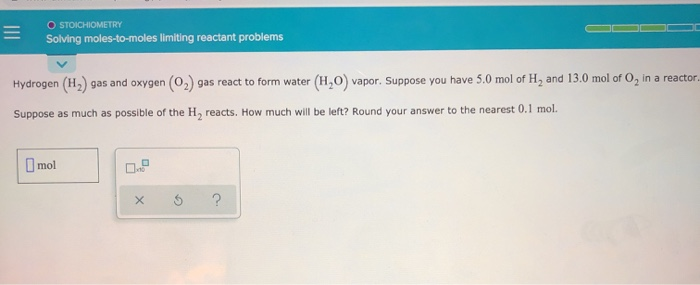O STOICHIOMETRY Solving moles-to-moles limiting reactant problems Hydrogen (H2) gas and oxygen (O2) gas react to form water (H,) vapor. Suppose you have 5.0 mol of H, and 13.0 mol of O, in a reactor Suppose as much as possible of the H, reacts. How much will be left? Round your answer to the nearest 0.1 mol. mol x ?

• ### Model 4: Using Moles of Limiting Reagent in Stoichiometry The above pictures are not realistic because...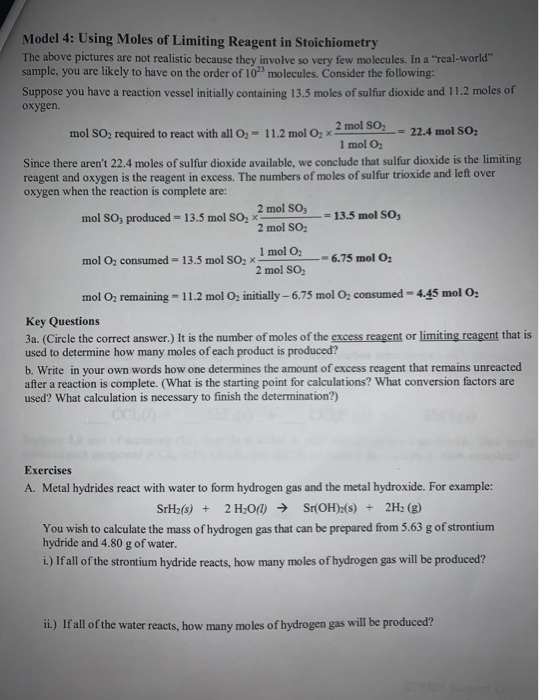Model 4: Using Moles of Limiting Reagent in Stoichiometry The above pictures are not realistic because they involve so very few molecules. In a "real-world" sample, you are likely to have on the order of 10 molecules. Consider the following: Suppose you have a reaction vessel initially containing 13.5 moles of sulfur dioxide and 11.2 moles of oxygen mol SO, required to react with all O2 = 11.2 mol O2 x 2 mol SO 22.4 mol SO, 1 mol O2...

• ### O STOICHIOMETRY Solving moles-to-moles limiting reactant problems - Hydrogen (H2) gas and oxygen (O2) gas react...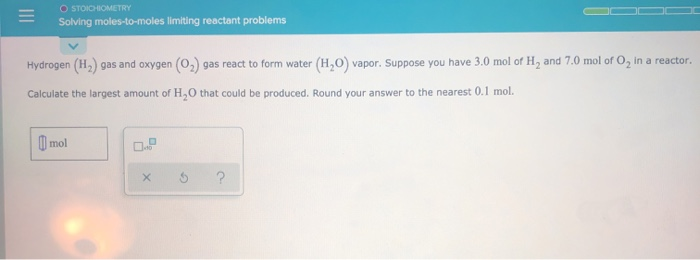O STOICHIOMETRY Solving moles-to-moles limiting reactant problems - Hydrogen (H2) gas and oxygen (O2) gas react to form water (H20) vapor. Suppose you have 3.0 mol of H, and 7.0 mol of o, in a reactor. Calculate the largest amount of H,O that could be produced. Round your answer to the nearest 0.1 mol. Il mol x 5 ?

• ### please answer both. I just need these two last questions and there due at midnight. Oxygen gas can be prepared by h...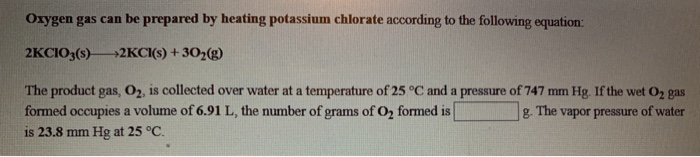please answer both. I just need these two last questions and there due at midnight. Oxygen gas can be prepared by heating potassium chlorate according to the following equation 2KCIO3(s) *2KCI(s) + 302(g) The product gas, O2, is collected over water at a temperature of 25 °C and a pressure of 747 mm Hg. If the wet O2 gas formed occupies a volume of 6.91 L, the number of grams of O, formed is g. The vapor pressure of water...

• ### Which of the following statements is false concerning the reaction of hydrogen gas and oxygen gas given below? H2(g)+1...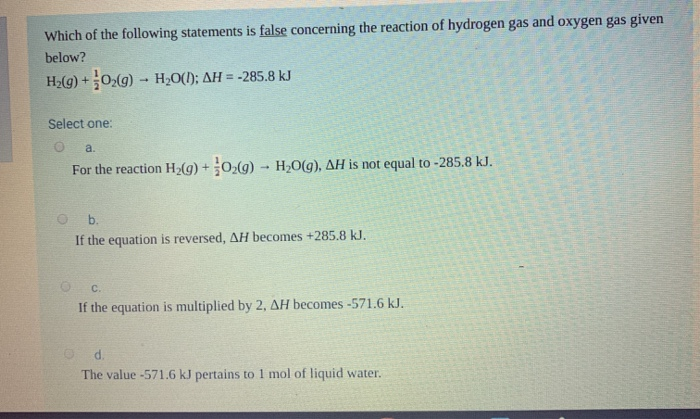Which of the following statements is false concerning the reaction of hydrogen gas and oxygen gas given below? H2(g)+1/2O2(g)>H2O Which of the following statements is false concerning the reaction of hydrogen gas and oxygen gas given below? H2(g) +029) - H20(1; AH = -285.8 kJ Select one: a. For the reaction H2(g) + O2(g) - H2O(g), AH is not equal to -285 b. If the equation is reversed, AH becomes +285.8 kJ. If the equation is multiplied by 2, AH...

• ### Consider the following gas reaction at STP: H2(g) +O2(g) -->H2O2(g). Calculate the final volume if 2.0...

Consider the following gas reaction at STP: H2(g) +O2(g) -->H2O2(g). Calculate the final volume if 2.0 moles of hydrogen gas react with 2.0 moles of oxygen gas. (R=0.08206 L*atm/mol*K)

• ### I also don’t know what the balanced equation is. I am not sure if my work...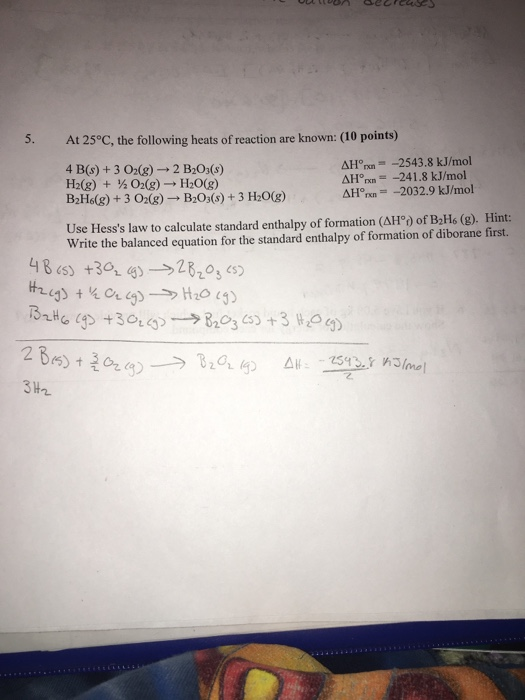I also don’t know what the balanced equation is. I am not sure if my work is right so please disregard what I have down at this moment. 5. At 25°C, the following heats of reaction are known: (10 points) 4 B(s) + 3 O2(g) → 2 B2O3(s) H2(g) + ½Oz(g) → H2O(g) B2H6(g) + 3 Odg) → B2O3(s) + 3 H2O(g) ΔHonn =-2543.8 kJ/mol ARI"nn=-241.8 kJ/mol AH n-2032.9 kJ/mol Use Hess's law to calculate standard enthalpy of formation (AH)...